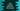# Java.util.Arrays.copyOf() method explanation with example## Java.util.Arrays.copyOf() method explanation with example:

copyOf method can be used to copy an array in Java. It takes one array and a length value as the parameters and copies that array.

In this post, we will learn how to use copyOf method with examples.

### Definition of copyOf:

copyOf is defined as like below:

``public static <T> T[] copyOf(T[] arr, int length)``

Here,

• arr is the original to copy from.
• length is the final length of the new array.

It returns a copy of the original array. If the length is smaller than the array length, it truncates the array. If it is bigger than the array length, it is padded with null to make the copy equal to the given length.

Other than that, both copies will have the same length. The result array class will be same as the given array.

### Exceptions:

It can throw any of the two exceptions:

• NegativeArraySizeException if the length is negative.
• NullPointerException if the array is null.

### Example of copyOf:

Let’s take an example of copyOf:

``````import java.util.Arrays;

class Main {
public static void printArray(int[] arr) {
for (int j : arr) {
System.out.println(j);
}
}

public static void main(String[] args) {
int[] givenArray = new int[]{1, 2, 3, 4, 5, 6, 7};

int[] copiedArray = Arrays.copyOf(givenArray, givenArray.length);
copiedArray = 0;
copiedArray = 1;

System.out.println("Given array: ");
printArray(givenArray);

System.out.println("Copied array: ");
printArray(copiedArray);
}
}``````

Here,

• printArray method is to print an array. It takes one integer array as the parameter and prints that array.
• givenArray is the original array of integers.
• We used Arrays.copyOf to create a copy of this array and assigned it to copiedArray. We are also changing the elements at 0th and 1st index of the array.
• Both arrays are printed at the end of the program.

It will print the below output:

``````Given array:
1
2
3
4
5
6
7
Copied array:
0
1
3
4
5
6
7``````

As you can see here, the items at 0th and 1st indices are changed for the copied array, but these are not changed for the original array, i.e. both are not referring to the same memory location.

### Example 2: If the final length is greater than the length of the original array:

If the final length is greater than the length of the original array, it will add null to fill the extra positions. Let’s check it with an example:

``````import java.util.Arrays;

class Main {
public static void printArray(String[] arr) {
for (String j : arr) {
System.out.println(j);
}
}

public static void main(String[] args) {
String[] givenArray = new String[]{"Zero", "One", "Two"};

String[] copiedArray = Arrays.copyOf(givenArray, givenArray.length + 5);

System.out.println("Given array: ");
printArray(givenArray);

System.out.println("Copied array: ");
printArray(copiedArray);
}
}``````

Here,

• We are using String arrays.
• The final length is 5 more than the array length.

It will print:

``````Given array:
Zero
One
Two
Copied array:
Zero
One
Two
null
null
null
null
null``````

As you can see, it added null for the extra elements. For an integer array, it will add 0.

### Example 3: If the final length is smaller than the length of the original array:

It is simple. If the final length is smaller than the length of the original array, it will copy till the length, i.e. it will not copy the full array. For example:

``````import java.util.Arrays;

class Main {
public static void printArray(char[] arr) {
for (char j : arr) {
System.out.println(j);
}
}

public static void main(String[] args) {
char[] givenArray = new char[]{'a', 'e', 'i', 'o', 'u'};

char[] copiedArray = Arrays.copyOf(givenArray, 2);

System.out.println("Given array: ");
printArray(givenArray);

System.out.println("Copied array: ");
printArray(copiedArray);
}
}``````

In this example, I am using a character array and the final length is less than the original array length. If you run this program, it will print:

``````Given array:
a
e
i
o
u
Copied array:
a
e``````

As you can see, it copied only the first two elements from the original array.

### Example of exceptions:

As I have explained before, it can throw either NegativeArraySizeException or NullPointerException.

If I pass any negative final length, it will throw NegativeArraySizeException as like below:

Or, if the array is null, it will throw NullPointerException:

You can wrap the code with a try-catch block to handle the exception:

``````try {
char[] copiedArray = Arrays.copyOf(givenArray, -1);
System.out.println("Given array: ");
printArray(givenArray);

System.out.println("Copied array: ");
printArray(copiedArray);
} catch (NegativeArraySizeException e) {
System.out.println("NegativeArraySizeException");
} catch (NullPointerException e) {
System.out.println("NullPointerException");
}``````